# Excel DATEDIF - calculate difference between two dates in days, weeks, months or years

In this tutorial, you will find a simple explanation of the Excel DATEDIF function and a few formula examples that demonstrate how to compare dates and calculate the difference in days, weeks, months or years.

Over the past few weeks, we investigated nearly every aspect of working with dates and times in Excel. If you have been following our blog series, you already know how to insert and format dates in your worksheets, how to calculate days, weeks, months and years as well as add and subtract dates.

In this tutorial, we will focus on calculating date difference in Excel and you will learn different ways to count the number of days, weeks, months and years between two dates.

#### Easily find difference between two dates in Excel

##### Get the result as a ready-made formula in years, months, weeks, or days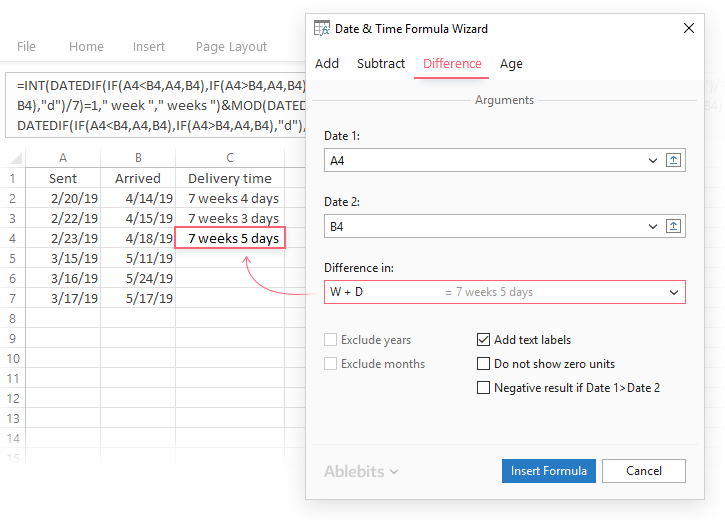Read more

#### Add and subtract dates in a couple of clicks

##### Delegate date & time formulas building to an expert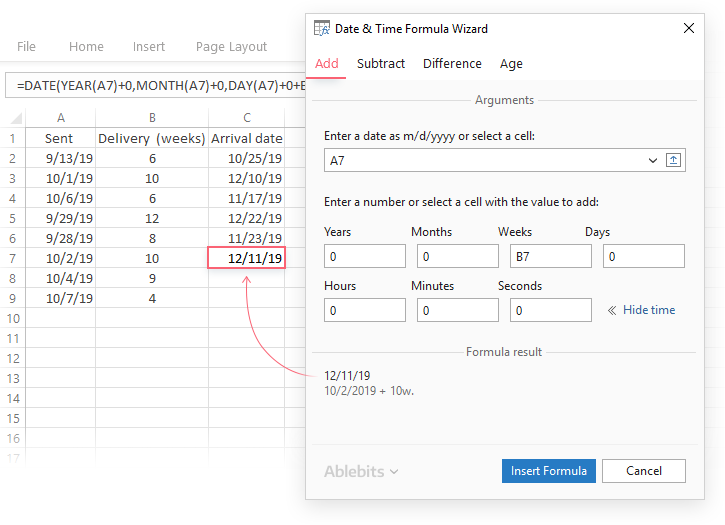Read more

#### Calculate age in Excel on the fly

##### And get a custom-tailored formula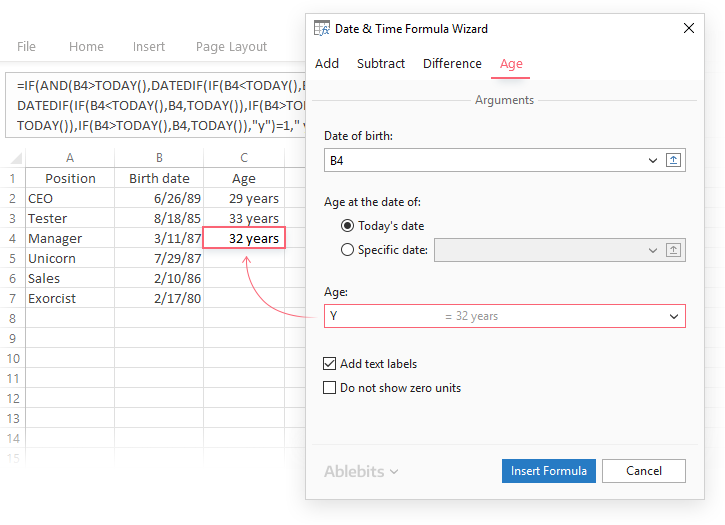Read more

## Excel DATEDIF function - calculating date difference

As its name suggests, the DATEDIF function is purposed for calculating the difference between two dates.

DATEDIF is one of very few undocumented functions in Excel, and because it is "hidden" you won't find it on the Formula tab, nor will you get any hint on which arguments to enter when you start typing the function's name in the formula bar. That is why it's important to know the complete syntax of Excel DATEDIF to be able to use it in your formulas.

#### Excel DATEDIF function - syntax

The syntax of the Excel DATEDIF function is as follows:

DATEDIF(start_date, end_date, unit)

All three arguments are required:

Start_date - the initial date of the period you want to calculate.

End_date - the ending date of the period.

To put it differently, start_date and end_date are two dates to calculate the difference between. In your DATEDIF formulas, you can input the dates is various ways, such as:

• Cell references. For example, the following formula counts the number of days between the dates in cells A1 and B1:

`=DATEDIF(A1, B1, "d")`

• Text strings. Excel understands dates in many formats, for example "20-May-2015", "5/20/2015", "2015/5/20", etc. The following formula calculates the number of months between the specified dates:

`=DATEDIF("5/10/2015", "7/20/2015", "m")`

• Serial numbers. Since Microsoft Excel stores each date as a serial number beginning with January 1, 1900, you can put numbers corresponding to the dates directly in the formula. Although fully supported, this method is not reliable because date numbering varies on different computer systems. In the 1900 date system, the following formula is another way to calculate months between two dates from the previous example (10-May-2015 and 20-Jul-2015):

`=DATEDIF(42134, 42205, "m")`

• Results of other functions. For instance, the following formula counts how many days there are between today's date and 20 May, 2015.

`=DATEDIF(TODAY(), "5/20/2015", "d")`

Note. In your formulas, the end date must always be greater than the start date, otherwise the Excel DATEDIF function returns the #NUM! error.

Unit - the time unit to use when calculating the difference between two dates. By supplying different units, you can get the DATEDIF function to return the date difference in days, months or years. Overall, 6 units are available, which are described in the following table.

 Unit Meaning Explanation Y Years Number of complete years between the start and end dates. M Months Number of complete months between the dates. D Days Number of days between the start date and end date. MD Days excluding years and months The date difference in days, ignoring months and years. YD Days excluding years The date difference in days, ignoring years. YM Months excluding days and years The date difference in months, ignoring days and years.

Hopefully, the above information has been helpful to understand the basics. And now, let's see how you can use the Excel DATEDIF function to compare dates in your worksheets and return the difference.

## How to get the number of days between two dates in Excel

If you observed DATEDIF's arguments carefully, you've noticed that there exist 3 different units for counting days between the dates. Which one to use depends on exactly what your needs are.

#### Example 1. Excel DATEDIF formula to calculate date difference in days

Supposing you have the start date in cell A2 and the end date in cell B2 and you want Excel to return the date difference in days. A simple DATEDIF formula works just fine:

`=DATEDIF(A2, B2, "d")`

Provided that a value in the start_date argument is less than in end_date. In case the start date is greater than the end date, the Excel DATEDIF function returns the #NUM error, as in row 5: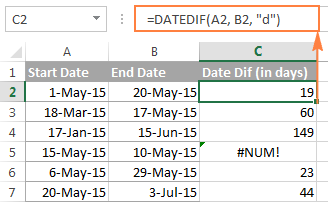If you are looking for a formula that can return the date difference in days as either a positive or negative number, simply subtract one date directly from the other:

`=B2-A2`

Please see How to subtract dates in Excel for full details and more formula examples.

#### Example 2. Count days in Excel ignoring years

Supposing you have two lists of dates that belong to different years and you wish to calculate the number of days between the dates as if they were of the same year. To do this, use a DATEDIF formula with "YD" unit:

`=DATEDIF(A2, B2, "yd")`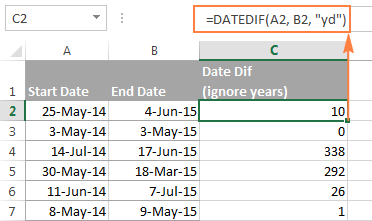If you want the Excel DATEDIF function to ignore not only years but also moths, then use the "md" unit. In this case, your formula will calculate days between two dates as if they were of the same month and the same year:

`=DATEDIF(A2, B2, "md")`

The screenshot below demonstrates the results, and comparing it with the screenshot above can help understand the difference better.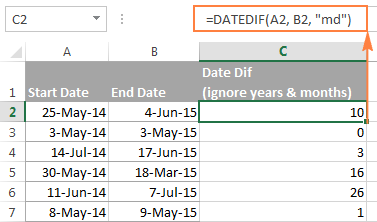Tip. To get the number of working days between two dates, use the NETWORKDAYS or NETWORKDAYS.INTL function.

## How to calculate date difference in weeks

As you probably noticed, the Excel DATEDIF function does not have a special unit to calculate date difference in weeks. However, there is an easy workaround.

To find out how many weeks there are between two dates, you can use the DATEDIF function with "D" unit to return the difference in days, and then divide the result by 7.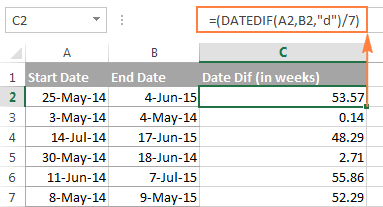To get the number of full weeks between the dates, wrap your DATEDIF formula in the ROUNDDOWN function, which always rounds the number towards zero:

`=ROUNDDOWN((DATEDIF(A2, B2, "d") / 7), 0)`

Where A2 is the start date and B2 is the end date of the period you are calculating.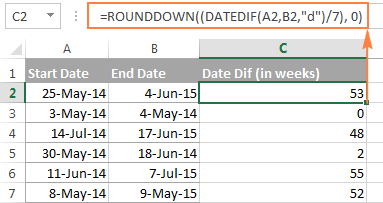## How to calculate the number of months between two dates in Excel

Similarly to counting days, the Excel DATEDIF function can compute the number of months between two dates that you specify. Depending on the unit you supply, the formula will produce different results.

#### Example 1. Calculate complete months between two dates (DATEDIF)

To count the number of whole months between the dates, you use the DATEDIF function with "M" unit. For example, the following formula compares the dates in A2 (start date) and B2 (end date) and returns the difference in months:

`=DATEDIF(A2, B2, "m")`

Note. For the DATEDIF formula to calculate months correctly, the end date should always be greater than the start date; otherwise the formula returns the #NUM error.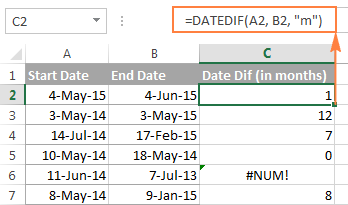To avoid such errors, you may force Excel to always perceive an older date as the start date, and a more recent date as the end date. To do this, add a simple logical test:

`=IF(B2>A2, DATEDIF(A2,B2,"m"), DATEDIF(B2,A2,"m"))`

#### Example 2. Get the number of months between two dates ignoring years (DATEDIF)

To count the number of months between the dates as if they were of the same year, type "YM" in the unit argument:

`=DATEDIF(A2, B2, "ym")`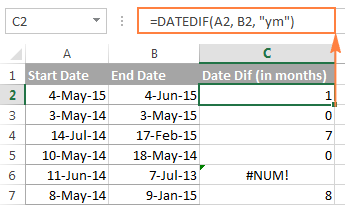As you see, this formula also returns an error in row 6 where end date is less than the start date. If your data set may contain such dates, you will find the solution in the next examples.

#### Example 3. Calculating months between two dates (MONTH function)

An alternative way to calculate the number of months between two dates in Excel is using the MONTH function, or more precisely a combination of MONTH and YEAR functions:

`=(YEAR(B2) - YEAR(A2))*12 + MONTH(B2) - MONTH(A2)`

Of course, this formula is not so transparent as DATEDIF and it does take time to wrap your head around the logic. But unlike the DATEDIF function, it can compare any two dates and return the difference in months as either a positive or negative value: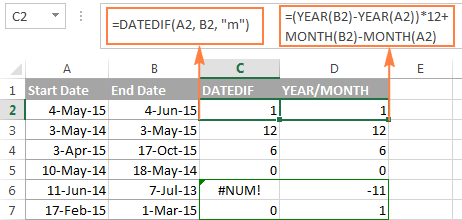Notice that the YEAR/MONTH formula has no problem with calculating months in row 6 where the start date is more recent than the end date, the scenario in which an analogues DATEDIF formula fails.

Note. The results returned by DATEDIF and YEAR/MONTH formulas are not always identical because they operate based on different principles. The Excel DATEDIF function returns the number of complete calendar months between the dates, while the YEAR/MONTH formula operates on months' numbers.

For example, in row 7 in the screenshot above, the DATEDIF formula returns 0 because a complete calendar month between the dates has not elapsed yet, while YEAR/MONTH returns 1 because the dates belong to different months.

#### Example 4. Counting months between 2 dates ignoring years (MONTH function)

In case all of your dates are of the same year, or you want to calculate months between the dates ignoring years, you can the MONTH function to retrieve the month from each date, and then subtract one month from the other:

`=MONTH(B2) - MONTH(A2)`

This formula works similarly to Excel DATEDIF with "YM" unit as demonstrated in the following screenshot: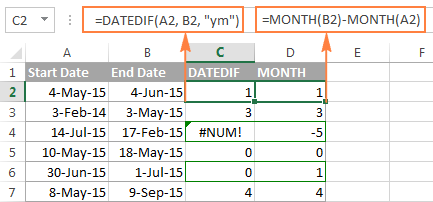However, the results returned by two formulas differ is a couple of rows:

• Row 4: the end date is less than the start date and therefore DATEDIF returns an error while MONTH-MONTH yields a negative value.
• Row 6: the dates are of different months, but the actual date difference is just one day. DATEDIF returns 0 because it calculates whole months between 2 dates. MONTH-MONTH returns 1 because it subtracts the months' numbers from each other ignoring days and years.

## How to calculate years between two dates in Excel

If you followed the previous examples where we calculated months and days between two dates, then you can easily derive a formula to calculate years in Excel. The following examples can help you check if you got the formula right :)

#### Example 1. Calculating complete years between two dates (DATEDIF function)

To find out the number of complete calendar years between two dates, use the old good DATEDIF with the "Y" unit:

`=DATEDIF(A2,B2,"y")`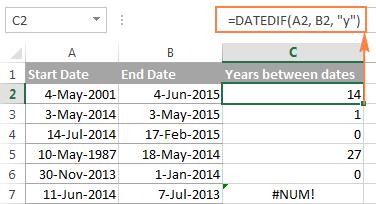Notice that the DATEDIF formula returns 0 in row 6, although the dates are of different years. This is because the number of full calendar years between the start and end dates equals to zero. And I believe you are not surprised to see the #NUM! error in row 7 where the start date is more recent than the end date.

#### Example 2. Calculating years between two dates (YEAR function)

An alternative way to calculate years in Excel is using the YEAR function. Similarly to the MONTH formula, you extract the year from each date, and then subtract the years from each other:

`=YEAR(B2) - YEAR(A2)`

In the following screenshot, you can compare the results returned by the DATEDIF and YEAR functions: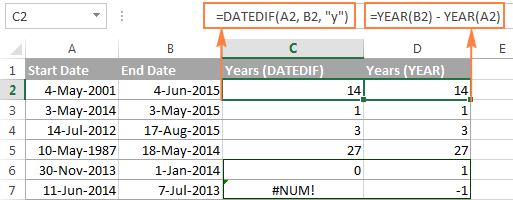In most cases the results are identical, except that:

• The DATEDIF function calculates complete calendar years, while the YEAR formula simply subtracts one year from the other. Row 6 illustrates the difference.
• The DATEDIF formula returns an error if the start date is greater than the end date, while the YEAR function returns a negative value, as in row 7.

## How to get date difference in days, months and years

To count the number of complete years, months and days between two dates in a single formula, you simply concatenate three DATEDIF functions:

`=DATEDIF(A2, B2, "y") &" years, "&DATEDIF(A2, B2, "ym") &" months, " &DATEDIF(A2, B2, "md") &" days"`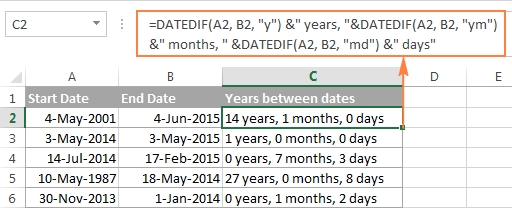If you'd rather not display zero values, you can wrap each DATEDIF in the IF function as follows:

`=IF(DATEDIF(A2,B2,"y")=0, "", DATEDIF(A2,B2,"y") & " years ") & IF(DATEDIF(A2,B2,"ym")=0,"", DATEDIF(A2,B2,"ym") & " months ") & IF(DATEDIF(A2, B2, "md")=0, "", DATEDIF(A2, B2, "md") & " days"`

The formula displays only non-zero elements as demonstrated in the following screenshot: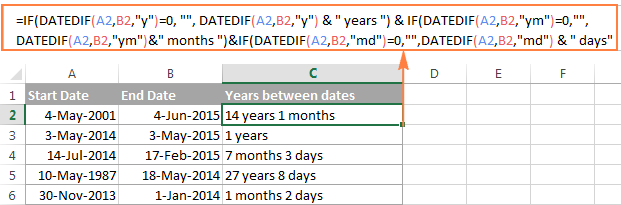## DATEDIF formulas to calculate age in Excel

In fact, calculating someone's age based on the date of birth is a special case of calculating date difference in Excel, where the end date is today's date. So, you use a usual DATEDIF formula with "Y" unit that returns the number of years between the dates, and enter the TODAY() function in the end_date argument:

`=DATEDIF(A2, TODAY(), "y")`

Where A2 is the birth date.

The above formula calculates the number of complete years. If you'd rather get the exact age, including years, months and days, then concatenate three DATEDIF functions like we did in the previous example:

`=DATEDIF(B2,TODAY(),"y") & " Years, " & DATEDIF(B2,TODAY(),"ym") & " Months, " & DATEDIF(B2,TODAY(),"md") & " Days"`

And you will get the following result: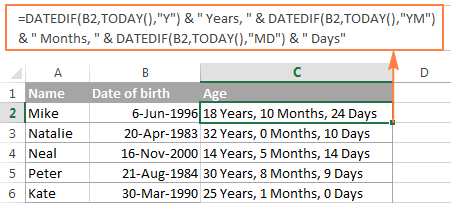To learn other methods of converting a birthdate to age, check out How to calculate age from date of birth.

## Date & Time Wizard - easy way to build date difference formulas in Excel

As demonstrated in the first part of this tutorial, Excel DATEDIF is quite a versatile function suitable for a variety of different uses. However, there is one significant drawback - it is undocumented by Microsoft, meaning, you won't find DATEDIF in the list of functions nor will you see any argument tooltips when you start typing a formula in a cell. To be able to use the DATEDIF function in your worksheets, you have to remember its syntax and enter all the arguments manually, which might be a time-consuming and error-prone way, especially for beginners.

Ultimate Suite for Excel changes this radically as it now provides the Date & Time Wizard that can make almost any date difference formula in no time. Here's how:

1. Select the cell where you want to insert the formula.
2. Go to the Ablebits Tools tab > Date & Time group, and click the Date & Time Wizard button: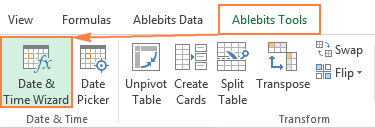3. The Date & Time Wizard dialog window shows up, you switch to the Difference tab and supply data for the formula arguments:
• Click in the Date 1 box (or click the Collapse Dialog button to the right of the box) and select a cell containing the first date.
• Click in the Date 2 box and select a cell with the second date.
• Select the desired unit or combination of units from the Difference in drop-down menu. As you do this, the wizard lets you preview the result in the box and the formula in the cell.
• If you are happy with the preview, click the Insert formula button, otherwise try different units.

For example, this is how you can get the number of days between two dates in Excel: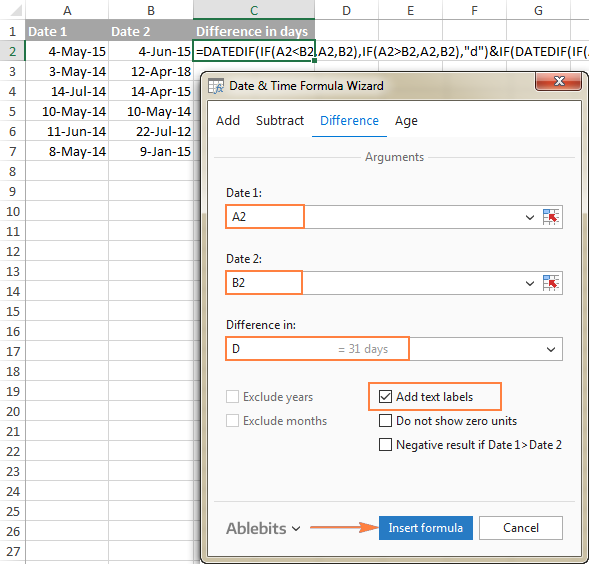Once the formula is inserted in the selected cell, you can copy it to other cells as usual by double-clicking or dragging the fill handle. The result will look similar to this: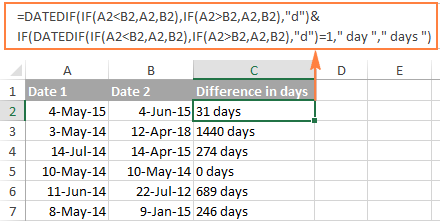To present the results in the most suitable way, a few more additional options are available:

• Exclude years and/or exclude months from calculations.
• Show or do not show text labels like days, months, weeks, and years.
• Show or do not show zero units.
• Return the results as negative values if Date 1 (start date) is greater than Date 2 (end date).

As an example, let's get the difference between two dates in years, months, weeks and days, ignoring zero units: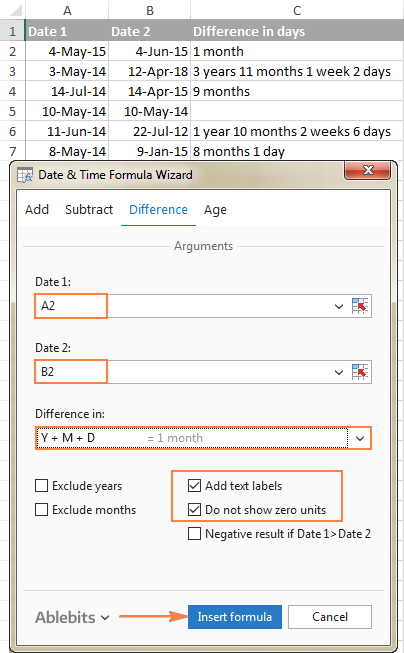### Benefits of using Date & Time Formula Wizard

Apart from speed and simplicity, the Date & Time Wizard provides a few more advantages:

• Unlike a regular DATEDIF formula, an advanced formula created by the wizard does not care which of the two dates is smaller and which is larger. The difference is always calculated perfectly even if Date 1 (start date) is greater than Date 2 (end date).
• The wizard supports all possible units (days, weeks, months and years) and lets you choose from 11 different combinations of these units.
• The formulas the wizard builds for you are normal Excel formulas, so you are free to edit, copy or move them as usual. You can also share your worksheets with other people, and all formulas will remain in place, even if someone does not have the Ultimate Suite in their Excel.

This is how you compute the difference between two dates in various time intervals. Hopefully, the DATEDIF function and other formulas you've learned today will prove useful in your work.